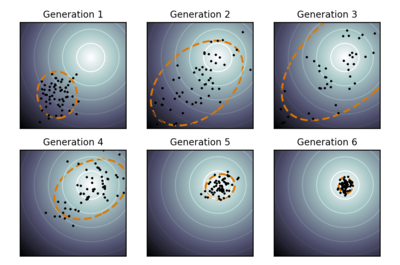# Covariance Matrix Adaptation Evolutionary Strategy (CMA-ES)

#include <pagmo/algorithms/cmaes.hpp>

class pagmo::cmaesCMA-ES is one of the most successful algorithm, classified as an Evolutionary Strategy, for derivative-free global optimization. The version implemented in PaGMO is the “classic” version described in the 2006 paper titled “The CMA evolution strategy: a comparing review.”.

Warning

A moved-from pagmo::cmaes is destructible and assignable. Any other operation will result in undefined behaviour.

Note

This user-defined algorithm is available only if pagmo was compiled with the PAGMO_WITH_EIGEN3 option enabled (see the installation instructions).

Note

Since at each generation all newly generated individuals sampled from the adapted distribution are reinserted into the population, CMA-ES may not preserve the best individual (not elitist). As a consequence the plot of the population best fitness may not be perfectly monotonically decreasing.

Hansen, Nikolaus. “The CMA evolution strategy: a comparing review.” Towards a new evolutionary computation. Springer Berlin Heidelberg, 2006. 75-102.

Public Types

typedef std::tuple<unsigned, unsigned long long, double, double, double, double> log_line_type

Single data line for the algorithm’s log.

A log data line is a tuple consisting of:

• the generation number,

• the number of function evaluations

• the best fitness vector so far,

• the population flatness evaluated as the distance between the decisions vector of the best and of the worst individual,

• the population flatness evaluated as the distance between the fitness of the best and of the worst individual.

typedef std::vector<log_line_type> log_type

Log type.

The algorithm log is a collection of cmaes::log_line_type data lines, stored in chronological order during the optimisation if the verbosity of the algorithm is set to a nonzero value (see cmaes::set_verbosity()).

Public Functions

cmaes(unsigned gen = 1, double cc = -1, double cs = -1, double c1 = -1, double cmu = -1, double sigma0 = 0.5, double ftol = 1e-6, double xtol = 1e-6, bool memory = false, bool force_bounds = false, unsigned seed = pagmo::random_device::next())

Constructor.

Constructs cmaes

Parameters
• gen – number of generations.

• cc – backward time horizon for the evolution path (by default is automatically assigned)

• cs – makes partly up for the small variance loss in case the indicator is zero (by default is automatically assigned)

• c1 – learning rate for the rank-one update of the covariance matrix (by default is automatically assigned)

• cmu – learning rate for the rank- $$\mu$$ update of the covariance matrix (by default is automatically assigned)

• sigma0 – initial step-size

• ftol – stopping criteria on the x tolerance (default is 1e-6)

• xtol – stopping criteria on the f tolerance (default is 1e-6)

• memory – when true the adapted parameters are not reset between successive calls to the evolve method

• force_bounds – when true the box bounds are enforced. The fitness will never be called outside the bounds but the covariance matrix adaptation mechanism will worsen

• seed – seed used by the internal random number generator (default is random)

Throws

std::invalid_argument – if cc, cs, c1 and cmu are not in [0, 1]

population evolve(population) const

Algorithm evolve method.

Evolves the population for a maximum number of generations, until one of tolerances set on the population flatness (x_tol, f_tol) are met.

Parameters

pop – population to be evolved

Throws
• std::invalid_argument – if the problem is multi-objective or constrained

• std::invalid_argument – if the problem is unbounded

• std::invalid_argument – if the population size is not at least 5

Returns

evolved population

void set_seed(unsigned)

Sets the seed.

Parameters

seed – the seed controlling the algorithm stochastic behaviour

inline unsigned get_seed() const

Gets the seed.

Returns

the seed controlling the algorithm stochastic behaviour

inline void set_verbosity(unsigned level)

Sets the algorithm verbosity.

Sets the verbosity level of the screen output and of the log returned by get_log(). level can be:

• 0: no verbosity

• >0: will print and log one line each level generations.

Example (verbosity 1):

Gen:      Fevals:          Best:            dx:            df:         sigma:
51           1000    1.15409e-06     0.00205151    3.38618e-05       0.138801
52           1020     3.6735e-07     0.00423372    2.91669e-05        0.13002
53           1040     3.7195e-07    0.000655583    1.04182e-05       0.107739
54           1060    6.26405e-08     0.00181163    3.86002e-06      0.0907474
55           1080    4.09783e-09    0.000714699    3.57819e-06      0.0802022
56           1100    1.77896e-08    4.91136e-05    9.14752e-07       0.075623
57           1120    7.63914e-09    0.000355162    1.10134e-06      0.0750457
58           1140    1.35199e-09    0.000356034    2.65614e-07      0.0622128
59           1160    8.24796e-09    0.000695454    1.14508e-07        0.04993

Gen, is the generation number, Fevals the number of function evaluation used, Best is the best fitness function currently in the population, dx is the norm of the distance to the population mean of the mutant vectors, df is the population flatness evaluated as the distance between the fitness of the best and of the worst individual and sigma is the current step-size

Parameters

level – verbosity level

inline unsigned get_verbosity() const

Gets the verbosity level.

Returns

the verbosity level

inline unsigned get_gen() const

Gets the generations.

Returns

the number of generations to evolve for

inline std::string get_name() const

Algorithm name.

One of the optional methods of any user-defined algorithm (UDA).

Returns

a string containing the algorithm name

std::string get_extra_info() const

Extra info.

One of the optional methods of any user-defined algorithm (UDA).

Returns

a string containing extra info on the algorithm

inline const log_type &get_log() const

Get log.

A log containing relevant quantities monitoring the last call to evolve. Each element of the returned std::vector is a cmaes::log_line_type containing: Gen, Fevals, Best, dx, df, sigma as described in cmaes::set_verbosity

Returns

an std::vector of cmaes::log_line_type containing the logged values Gen, Fevals, Best, dx, df, sigma